### Home > PC > Chapter 1 > Lesson 1.1.3 > Problem1-39

1-39.
1. Use the idea of an inverse to find the missing angles in each triangle. Homework Help ✎

1.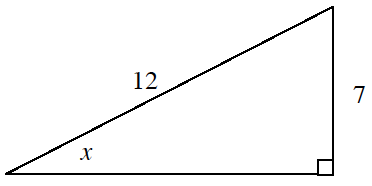2.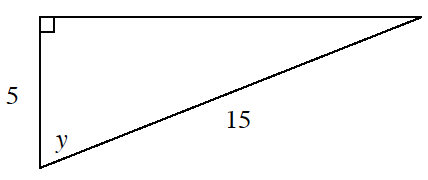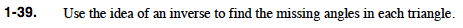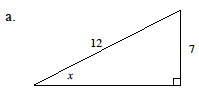$\text{sin}(x) = \frac{7}{12}$

Use the inverse sine button on your calculator.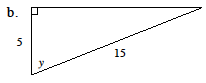Follow the same procedure your used part (a), but this time you will need to use a different trigonometric ratio.